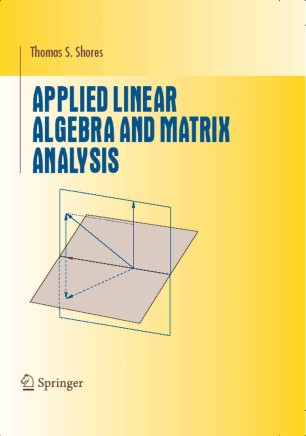# Applied Linear Algebra and Matrix Analysis

• Thomas S. ShoresTextbook

Part of the Undergraduate Texts in Mathematics book series (UTM)

1. Front Matter
Pages i-xii
2. Pages 1-54
3. Pages 55-144
4. Pages 145-210
5. Pages 211-249
6. Pages 251-304
7. Pages 305-354
8. Back Matter
Pages 355-383

### Introduction

This new book offers a fresh approach to matrix and linear algebra by providing a balanced blend of applications, theory, and computation, while highlighting their interdependence. Intended for a one-semester course, Applied Linear Algebra and Matrix Analysis places special emphasis on linear algebra as an experimental science, with numerous examples, computer exercises, and projects. While the flavor is heavily computational and experimental, the text is independent of specific hardware or software platforms.

Throughout the book, significant motivating examples are woven into the text, and each section ends with a set of exercises. The student will develop a solid foundation in the following topics

*Gaussian elimination and other operations with matrices

*basic properties of matrix and determinant algebra

*standard Euclidean spaces, both real and complex

*geometrical aspects of vectors, such as norm, dot product, and angle

*eigenvalues, eigenvectors, and discrete dynamical systems

*general norm and inner-product concepts for abstract vector spaces

For many students, the tools of matrix and linear algebra will be as fundamental in their professional work as the tools of calculus; thus it is important to ensure that students appreciate the utility and beauty of these subjects as well as the mechanics. By including applied mathematics and mathematical modeling, this new textbook will teach students how concepts of matrix and linear algebra make concrete problems workable.

Thomas S. Shores is Professor of Mathematics at the University of Nebraska, Lincoln, where he has received awards for his teaching. His research touches on group theory, commutative algebra, mathematical modeling, numerical analysis, and inverse theory.

### Keywords

Eigenvalue Eigenvector Matrix algebra linear algebra

#### Authors and affiliations

• Thomas S. Shores
• 1